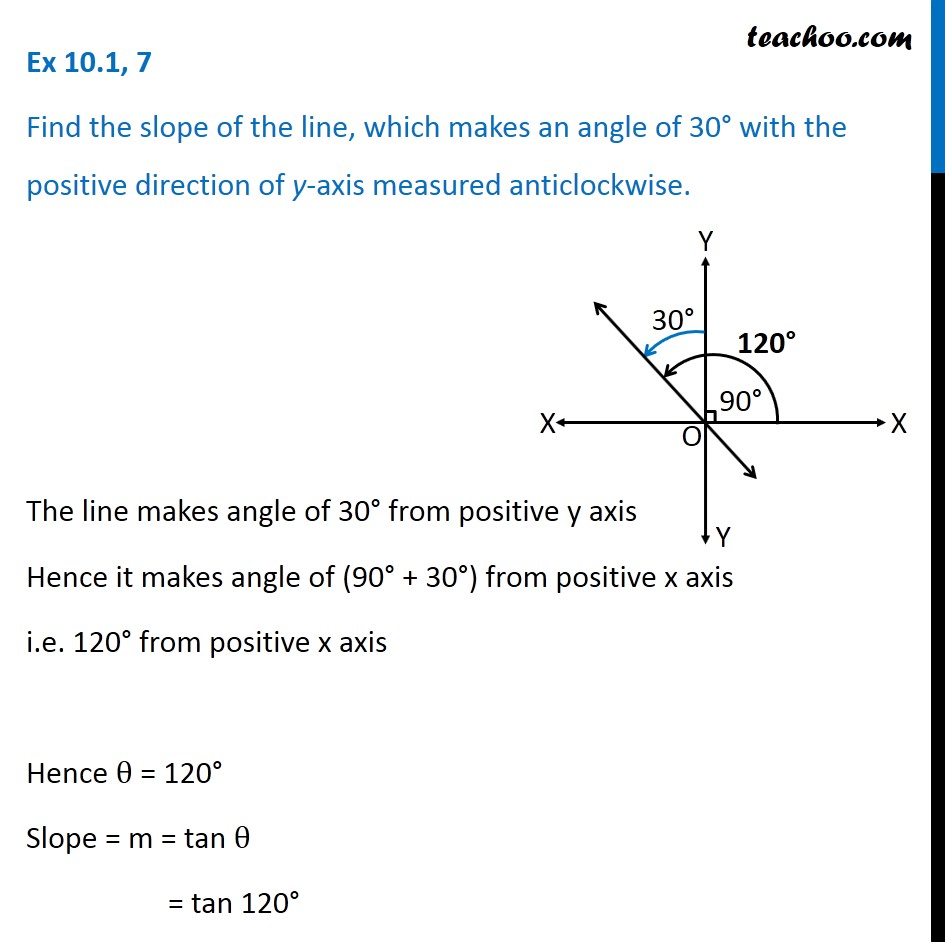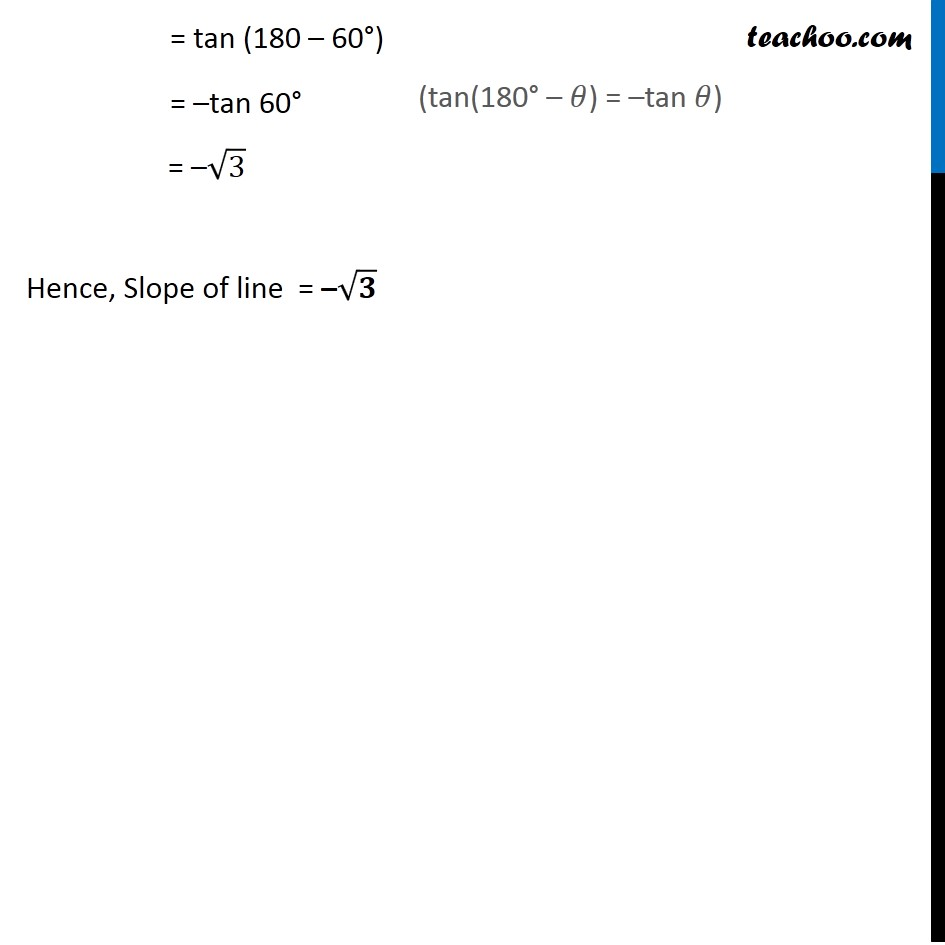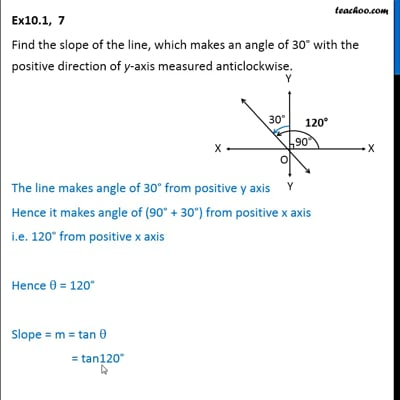Ex 10.1

Chapter 10 Class 11 Straight Lines (Term 1)
Serial order wiseThis video is only available for Teachoo black users

### Transcript

Ex 10.1, 7 Find the slope of the line, which makes an angle of 30° with the positive direction of y-axis measured anticlockwise. The line makes angle of 30° from positive y axis Hence it makes angle of (90° + 30°) from positive x axis i.e. 120° from positive x axis Hence θ = 120° Slope = m = tan θ = tan 120° = tan (180 – 60°) = –tan 60° = –√3 Hence, Slope of line = –√𝟑 (tan(180° – 𝜃) = –tan 𝜃)